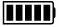ECE4253 Digital Communications Department of Electrical and Computer Engineering - University of New Brunswick, Fredericton, NB, Canada

# Galois Field GF(2) Calculator

 Model C-172 POLYNOMIAL CALCULATORA:

B:

 A + B A - B A × B A / B

Discussion  Polynomials  MATLAB

``` 110010000
----------- = 100100, Remainder = 100
1101```

## Detailed Calculation

 ``` 100100 ----------- 1101 ) 110010000 1101 ---- 00110000 1101 ---- 00100```

# MATLAB Example

Calculations in GF(2) are performed by MATLAB functions found within the Communications Toolbox.

>> a = gf( [1 1 0 0 1 0 0 0 0] );

>> b = gf( [1 1 0 1] );

>> [q,r] = deconv(a,b) % deconvolution is equivalent to division

q = GF(2) array.

Array elements =

1      0      0      1      0      0

r = GF(2) array.

Array elements =

1      0      0

 2020-02-22 14:07:48 AST Last Updated: 2010-04-29 Richard Tervo [ tervo@unb.ca ]